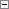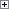Inventory valuation is the determination of the cost that is assigned to an inventory item, as expressed by the following equation.

Ending inventory = beginning inventory + net purchases - cost of goods sold

The calculation of inventory valuation uses the Cost Amount (Actual) field of the value entries for the item. The entries are classified according to the entry type that corresponds to the cost components, direct cost, indirect cost, variance, revaluation, and rounding. For more information, see Design Details: Cost Components.

Entries are applied against each other, either by the fixed application, or according to the general cost-flow assumption defined by the costing method. One entry of inventory decrease can be applied to more than one increase entry with different posting dates and possibly different acquisition costs. For more information, see Design Details: Item Application. Therefore, calculation of the inventory value for a given date is based on summing up positive and negative value entries.

Inventory Valuation report

To calculate the inventory value in the Inventory Valuation report, the report begins by calculating the value of the item’s inventory at a given starting date. It then adds the value of inventory increases and subtracts the value of inventory decreases up to a given ending date. The end result is the inventory value on the ending date. The report calculates these values by summing the values in the Cost Amount (Actual) field in the value entries, using the posting dates as filters.

The printed report always shows actual amounts, that is, the cost of entries that have been posted as invoiced. The report will also print the expected cost of entries that have posted as received or shipped, if you select the Include Expected Cost field on the Options FastTab.Important
Values in the Inventory Valuation report is reconciled with the Inventory account in the general ledger, meaning the value entries in question have been posted to the general ledger.Important
Amounts in the Value columns of the report are based on the posting date of transactions for an item.

Inventory Valuation - WIP report

A manufacturing company needs to determine the value of three types of inventory:

• Raw Materials inventory
• WIP inventory
• Finished Goods inventory

The value of WIP inventory is determined by the following equation.

Ending WIP inventory = Beginning WIP inventory + manufacturing costs - cost of goods manufactured

As for purchased inventory, the value entries provide the basis of the inventory valuation. The calculation is made using the values in the Cost Amount (Actual) field of the item and capacity value entries associated with a production order.

The purpose of WIP inventory valuation is to determine the value of the items whose manufacturing has not yet been completed on a given date. Therefore the WIP inventory value is based on the value entries related to the consumption and capacity ledger entries. Consumption ledger entries must be completely invoiced at the date of the valuation. Therefore, the Inventory Valuation - WIP report shows the costs representing the WIP inventory value in two categories: consumption and capacity.